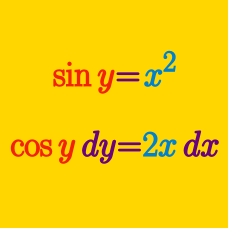Calculus

# Implicit Differentiation - Rational functions

If $\frac { x ( x - 6y) } { 5 - 4 y^ 2 } = 1,$ what is $$\displaystyle \frac{dy}{dx}?$$

If $$\frac{ 3 x ^2 + 7 xy^2 } { 8 + 5 y^3 } = 1$$, what is $$\displaystyle \frac{dy}{dx}?$$

If point $$(1,a)$$ with $$a>0$$ lies on the curve $\frac{y}{x}-\frac{9x}{y}=8,$ what is the derivative $$\displaystyle \frac{dy}{dx}$$ of the curve at the point?

Given $\frac{(x^2+y^2-11x)^2}{x^2+y^2}=4,$ what is the value of $$\displaystyle \frac{dy}{dx}$$ when $$x=0$$ and $$y=2 ?$$

If $\frac{6x-y^3}{y+x^2}=x+4,$ what is $$\displaystyle \frac{dy}{dx}?$$

×